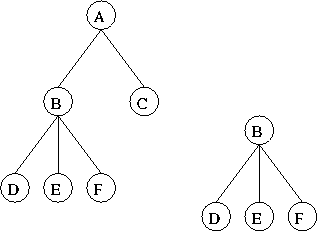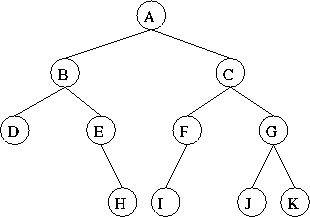Tom Kelliher, CS23

May 7, 1997

# Nomenclature

A tree:1. Vertex/node , directed edge.
2. Root, interior vertex, leaf.
3. Parent, child; ancestor, descendant.
4. Siblings.
5. Subtrees.
6. General trees.
7. A Binary tree, T, is a set of nodes such that either:
• T is empty.
• T is partitioned into three sets:
1. A single root.
2. Two possibly empty sets that are binary trees, the left and right subtrees of the root.
8. Binary search tree:
• Each vertex has a label. Consider a node labeled r:
1. If q is in r's left subtree, q is less than r.
2. If q is in r's right subtree, q is greater than r.
9. Height, level.
10. Full, complete. The book's defn. of complete is wrong. Counterexample?
11. Balanced binary tree, completely balanced binary tree.

Recursive structure.

• CreateBinaryTree()
• CreateBinaryTree(RootItem)
• CreateBinaryTree(RootItem, LeftTree, RightTree)
• DestroyBinaryTree()
• RootData()
• SetRootData(NewItem)
• AttachLeft(NewItem, Success)
• AttachRight(NewItem, Success)
• AttachLeftSubtree(LeftTree, Success)
• AttachRightSubtree(RightTree, Success)
• DetachLeftSubtree(LeftTree, Success)
• DetachRightSubtree(RightTree, Success)
• LeftSubtree()
• RightSubtree()
• PreorderTraverse(Visit)
• InorderTraverse(Visit)
• PostorderTraverse(Visit)

# Traversals

```typedef char NodeType;

struct NodeItem
{
NodeItem* left, right;
NodeType item;
};

typedef NodeItem* Node;
```

## Preorder

```void Preorder(Node T, void (*Visit)(NodeType))
{
if (T != NULL)
{
Visit(T->item);
Preorder(T->left, Visit);
Preorder(T->right, Visit);
}
}
```

## Inorder

```void Preorder(Node T, void (*Visit)(NodeType))
{
if (T != NULL)
{
Inorder(T->left, Visit);
Visit(T->item);
Inorder(T->right, Visit);
}
}
```

## Postorder

```void Preorder(Node T, void (*Visit)(NodeType))
{
if (T != NULL)
{
Postorder(T->left, Visit);
Postorder(T->right, Visit);
Visit(T->item);
}
}
```

## Examples

```void visit(NodeType n)
{
cout << n << endl;
}
```

Assume each function is called on the root of:What is printed?

## Pointer-Based Implementation

Been there, done that.

How do we get to the parent from the child?

## Array-Based Implementation

• Tree must be complete.
• Must know number of vertices.

The tree:

```char tree[MAXTREE];   // tree is root
```

children of `tree[i]`:

• Left child: `tree[2 * i + 1]`
• Right child: `tree[2 * i + 2]`

Can get to parent from child.

Thomas P. Kelliher
Tue May 6 15:54:24 EDT 1997
Tom Kelliher